SAT数学：代数例题 12

2019-09-21 SAT-数学 阅读

SAT Question Rank: Medium Topic: Algebra, Finding a constant in a system of equations

Below is a question from a recent SAT exam. It is a non-calculator question that would typically take a student 1-2 minutes to complete.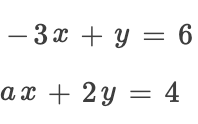In the system of equations above, a is a constant. For which of the following values of a does the system have no solution?
(A) –6
(B) –3
(C) 3
(D) 6

To answer this question, we need to manipulate both equations to look as similar as possible. We will start by making y the subject of both equations.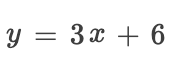And;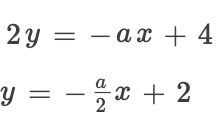Now we have our two equations, we can compare them. As they are both linear, they will not meet if they are parallel, i.e. have the same slope but different intercepts. This is the case if they have the same x coefficient but different constants.
To make this the case.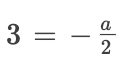So we solve this.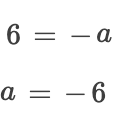Therefore, the answer is D, -6.• 地址：401-5811 Cooney Rd, Richmond, BC
• 地址：3030-8171 Ackroyd Rd, Richmond, BC
• 客服热线：604-370-0579 & 604-370-2569
• 联系邮箱：service@forbest.com

• 地址：江苏省无锡市滨湖区绣溪路50号K-PARK商务大楼3号楼3楼317室
• 客服热线：181-1861-8855

•官方公众号
•加拿大官方客服
•中国官方客服